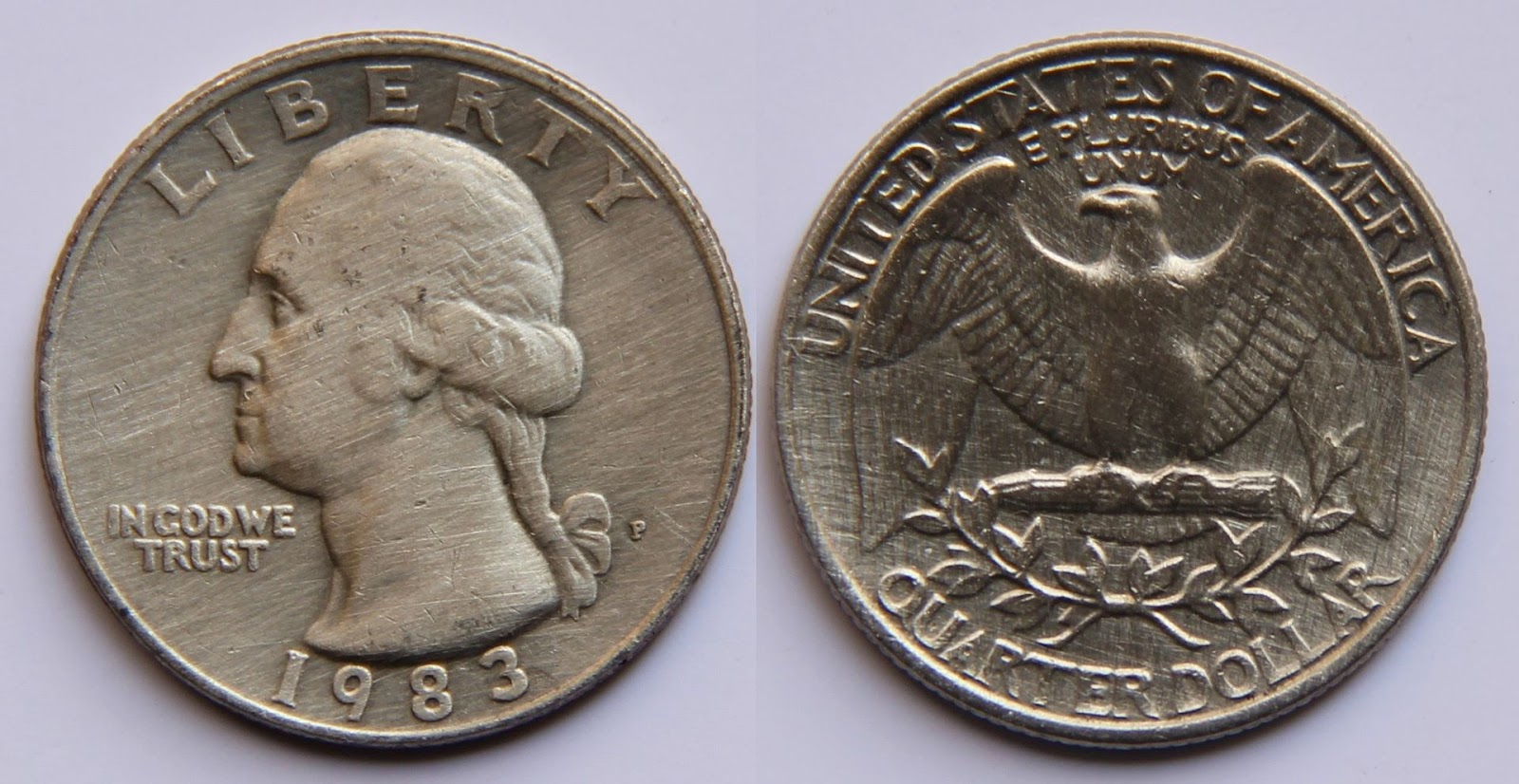## Saturday, January 2, 2021

Published January 02, 2021 by with 4 comments

# How Should You Bet on a Biased Coin Toss?

If you know a coin is biased to come up heads 75% of the time, what betting strategy should you use to bet on the outcome of a flip?What might seem intuitive is to have a mixed strategy of 75% heads and 25% tails. Maybe something like 'flip an unbiased coin twice and bet tails if you get tails twice and heads for any other outcome'. What result will that give you?

There are four possibilities here:

1. Coin lands on heads and you bet heads (75%*75% = 56.25% of the time)
2. Coin lands on heads and you bet tails (75%*25% = 18.75% of the time)
3. Coin lands on tails and you bet heads (25%*75% = 18.75% of the time)
4. Coin lands on tails and you bet tails (25%*25% = 6.25% of the time)
1 and 4 are winning situations, so you'll win 62.5% of the time this way (just sum the 1 and 4 win rates).

You might immediately notice that 62.5% is less than 75%. What if you just always bet heads? Filling out the same list as above:
1. Coin lands on heads and you bet heads (75%*100% = 75% of the time)
2. Coin lands on heads and you bet tails (75%*0% = 0% of the time)
3. Coin lands on tails and you bet heads (25%*100% = 25% of the time)
4. Coin lands on tails and you bet tails (25%*0% = 0% of the time)
1 and 4 are winning situations, so you'll win 75% of the time this way. In this situation, the general win rate is:

(coin bias*head bet percentage) + [(1 - coin bias)*(1 - head bet percentage)] = win rate

We want to maximize this. Using b for 'coin bias' and h for 'heat bet percentage':

b*h + (1 - b)*(1 - h) = win rate

b*h + 1 - h - b + b*h = win rate

2*b*h + 1 - h - b = win rate

h*(2*b - 1) + 1 - b = win rate

At this point, we have an equation for a line. Win rate vs h is a line with a slope of 2*b - 1 and an x-intercept of 1 - b. Anytime 2*b - 1 is positive, this line will go up and to the right so h = 1 is the best bet (heads 100% of the time). Anytime 2*b - 1 is negative, h = 0 is the best bet (tails 100% of the time). 2*b - 1 is positive whenever b is greater than 0.5. Thus, the optimal strategy here is bet in the direction of the bias 100% of the time when you have a known, biased coin.

1.⚡️✳️CASINO Online💵 💲คาสิโนสด ชั้นนำระดับโลก
♠️ บาคาร่า 🐡ยิงปลา 🦀น้ำเต้า
🎲ไฮโล ⚽️ บอล 🏟️ กีฬา 🎱 คาสิโน

👍เว็บตรงไม่ผ่านเอเจ้นท์ ฝาก•ถอน ระบบ𝗔𝗨𝗧𝗢💸
➡️ฝากขั้นต่ำ 1 บาท
➡️ถอนไม่จำกัดครั้ง 💯
➡️มีแอดมินดูแล24ชั่วโมง
➡️ไม่ต้องโยกเงินให้เสียเวลา💸
➡️รับทุกธนาคาร 🏧

2.It's tax-exempt - When you purchase or sell shares, get compensated profits or get revenue from a bank you should pay charges like stamp obligationlogin sbobet

3.This comment has been removed by the author.

4.Their poker instruction currently takes on another measurement requiring further developed technique and concentration.poker qq terpercaya